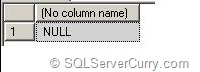### SQL Server: Handling Divide By Zero Error

Often SQL developers encounter "Divide by zero" error. This is because in an arithmetic
expression, when the divisor is zero (0), the expression throws an error. If you want to simply return NULL instead of an error, you can use one of the following methods

Method 1 : Use CASE expression

declare @var1 int ,@var2 int
select @var1=763, @var2=0

select @var1/case when @var2 =0 then null else @var2 end

OUTPUTMethod 2 : Use NULLIF function

declare @var1 int ,@var2 int
select @var1=763, @var2=0

select @var1/nullif(@var2,0)

The NULLIF function compares the first parameter (var2) with second parameter (0). If they are same, it returns a NULL